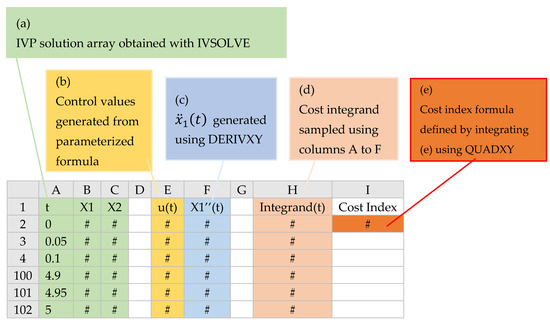Next Article in Journal / Special Issue
Differential Evolution Algorithm for Multilevel Assignment Problem: A Case Study in Chicken Transportation
Previous Article in Journal
Numerical Solution of Stochastic Generalized Fractional Diffusion Equation by Finite Difference Method
Previous Article in Special Issue
Optimal Control and Computational Method for the Resolution of Isoperimetric Problem in a Discrete-Time SIRS System

## Printed Edition

A printed edition of this Special Issue is available at MDPI Books....
Article

# Rapid Solution of Optimal Control Problems by a Functional Spreadsheet Paradigm: A Practical Method for the Non-Programmer

ExcelWorks LLC, Sharon, MA 02067, USA
Math. Comput. Appl. 2018, 23(4), 54; https://doi.org/10.3390/mca23040054
Received: 29 August 2018 / Revised: 24 September 2018 / Accepted: 26 September 2018 / Published: 28 September 2018
We devise a practical and systematic spreadsheet solution paradigm for general optimal control problems. The paradigm is based on an adaptation of a partial-parametrization direct solution method which preserves the original mathematical optimization statement, but transforms it into a simplified nonlinear programming problem (NLP) suitable for Excel NLP solver. A rapid solution strategy is implemented by a tiered arrangement of pure elementary calculus functions in conjunction with Excel NLP solver. With the aid of the calculus functions, a cost index and constraints are represented by equivalent formulas that fully encapsulate an underlining parametrized dynamical system. Excel NLP solver is then employed to minimize (or maximize) the cost index formula, by varying decision parameters, subject to the constraints formulas. The paradigm is demonstrated for several fixed and free-time nonlinear optimal control problems involving integral and implicit dynamic constraints with direct comparison to published results obtained by fundamentally different methods. Practically, applying the paradigm involves no more than defining a few formulas using basic Excel spreadsheet skills. View Full-Text
Show FiguresFigure 1

MDPI and ACS Style

Ghaddar, C.K. Rapid Solution of Optimal Control Problems by a Functional Spreadsheet Paradigm: A Practical Method for the Non-Programmer. Math. Comput. Appl. 2018, 23, 54. https://doi.org/10.3390/mca23040054

AMA Style

Ghaddar CK. Rapid Solution of Optimal Control Problems by a Functional Spreadsheet Paradigm: A Practical Method for the Non-Programmer. Mathematical and Computational Applications. 2018; 23(4):54. https://doi.org/10.3390/mca23040054

Chicago/Turabian Style

Ghaddar, Chahid K. 2018. "Rapid Solution of Optimal Control Problems by a Functional Spreadsheet Paradigm: A Practical Method for the Non-Programmer" Mathematical and Computational Applications 23, no. 4: 54. https://doi.org/10.3390/mca23040054

Find Other Styles
Note that from the first issue of 2016, MDPI journals use article numbers instead of page numbers. See further details here.

1